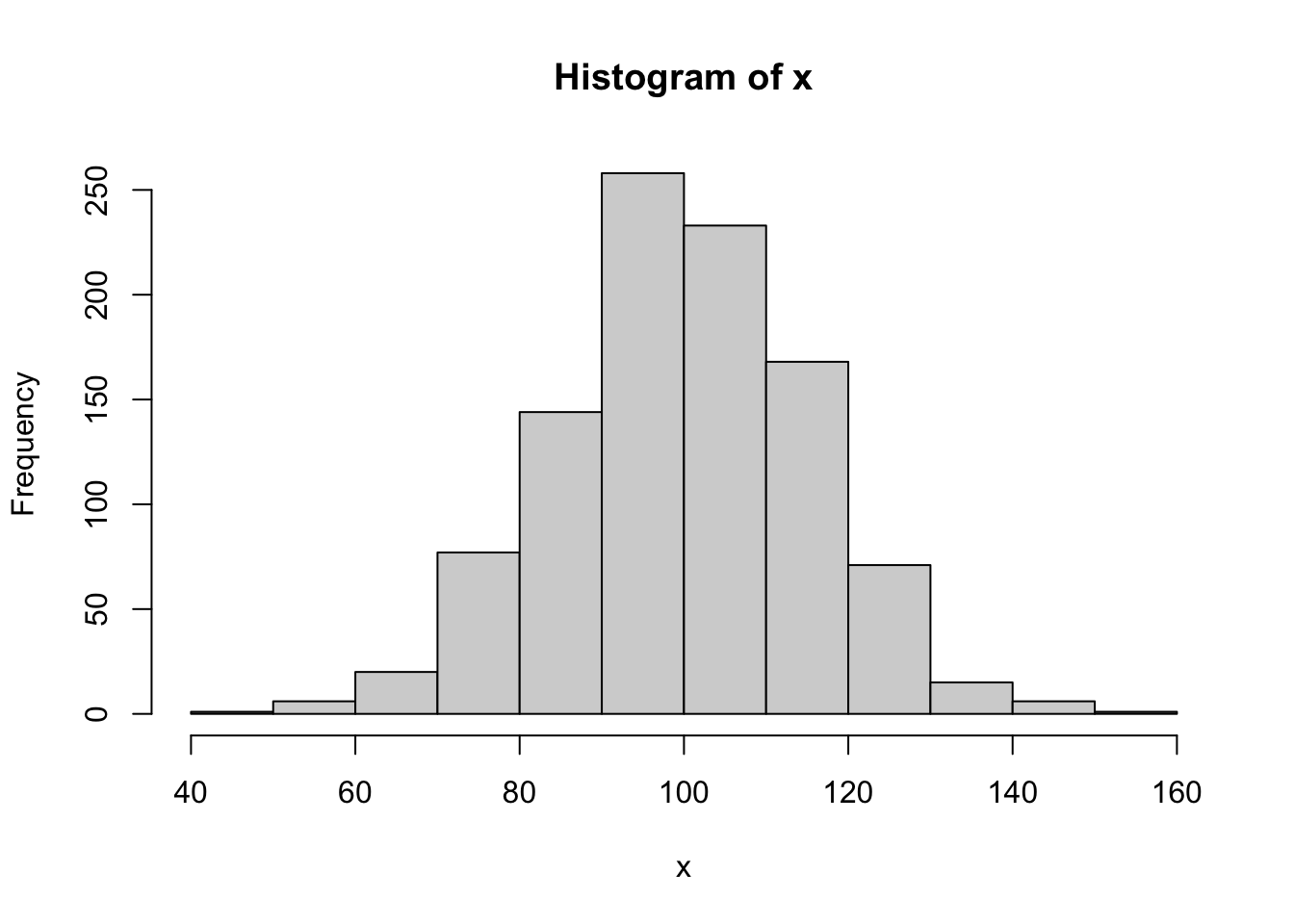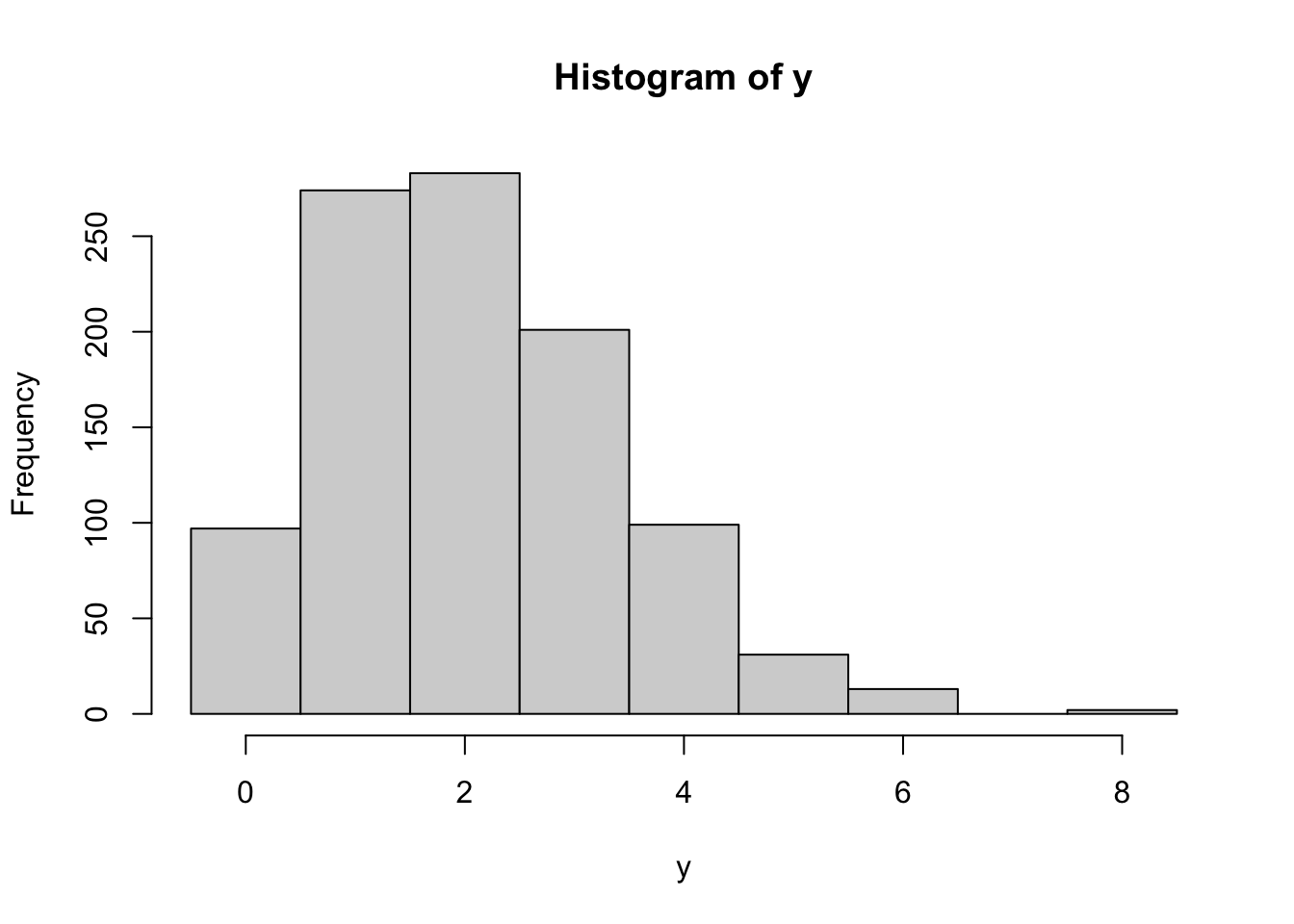The rand_bytes function binds to RAND_bytes in OpenSSL to generate cryptographically strong pseudo-random bytes. See the OpenSSL documentation for what this means.

rnd <- rand_bytes(10)
print(rnd)
  bc 17 8a 75 3f 55 a0 05 97 67

Bytes are 8 bit and hence can have 2^8 = 256 possible values.

as.numeric(rnd)
  188  23 138 117  63  85 160   5 151 103

Each random byte can be decomposed into 8 random bits (booleans)

x <- rand_bytes(1)
as.logical(rawToBits(x))
  TRUE  TRUE FALSE  TRUE FALSE  TRUE FALSE FALSE

## Secure Random Numbers

rand_num is a simple (2 lines) wrapper to rand_bytes to generate random numbers (doubles) between 0 and 1.

rand_num(10)
  0.1535481 0.5023007 0.8202547 0.6546440 0.4158769 0.4949917 0.4841464
 0.9379224 0.3792755 0.6939537

To map random draws from [0,1] into a probability density, we can use a Cumulative Distribution Function. For example we can combine qnorm and rand_num to simulate rnorm:

# Secure rnorm
x <- qnorm(rand_num(1000), mean = 100, sd = 15)
hist(x)Same for discrete distributions:

# Secure rbinom
y <- qbinom(rand_num(1000), size = 20, prob = 0.1)
hist(y, breaks = -.5:(max(y)+1))